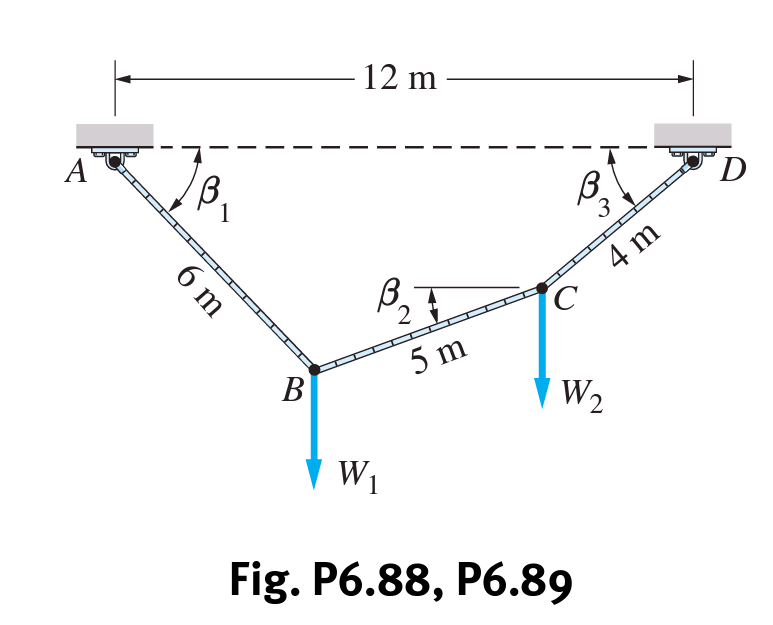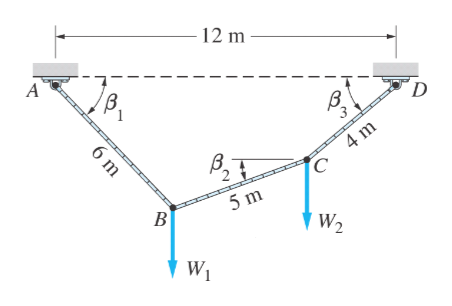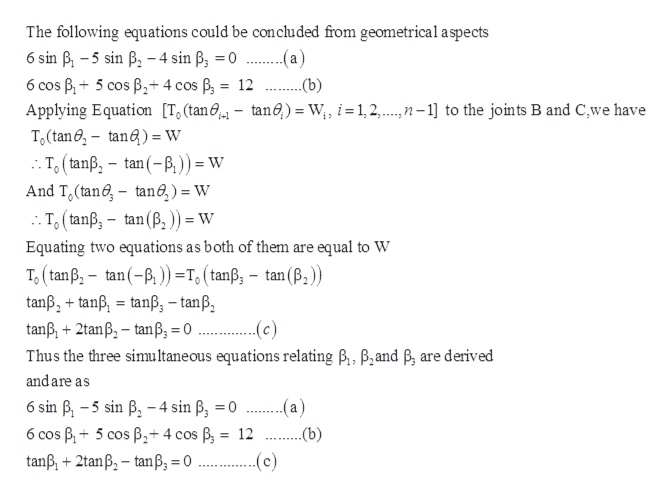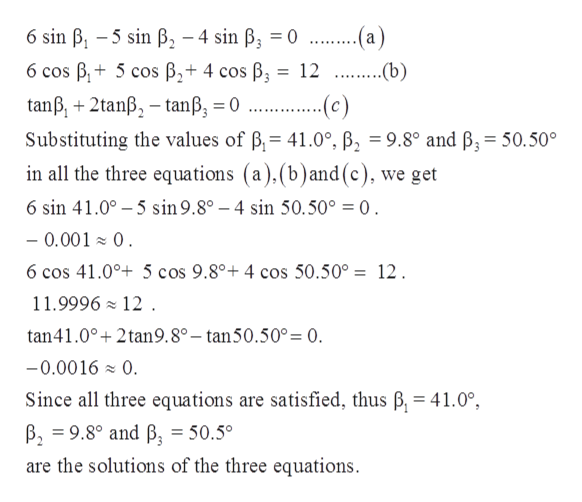# 12 mABВ,34 mВ,25 mBW2Fig. P6.88, P6.896 m

Question
3 views

Thecable of length 15m supports the forces W1 = W2 = W at B and C. (a) Derive the simultaneous equations for β1, β2, and β3. (b) Show that the solution to these equations is β1 = 41.0◦, β2 = 9.8◦, and β3 = 50.5◦. (c) Compute the force in each segment in terms of W.help_outlineImage Transcriptionclose12 m A B В, 3 4 m В, 2 5 m B W2 Fig. P6.88, P6.89 6 m fullscreen
check_circle

Step 1

Following is the given information:

The cable of length 15m supports the forces W1 = W2 = W at B and C.

Part (a).  Derive the simultaneous equations for β1, β2, and β3.

Part (b).  Show that the solution to these equations is β1 = 41.0◦, β2 = 9.8◦, and β3 = 50.5◦.

Part (c).  Compute the force in each segment in terms of W.help_outlineImage Transcriptionclose12 m A В. 'D В. 4m C 5 m В W2 6 m fullscreen
Step 2

Part A: Derive the simultaneous equations for β1, β2, and β3.

From the geometry  of the given figure first two equations will be deduced and third equation will be deduced from  the equation mentioned in the calculationhelp_outlineImage TranscriptioncloseThe following equations could be concluded from geometrical aspects 6 sin B -5 sin B4 sin B, 0 a) 6 cos B5 cos B2+ 4 cos B = 12 (b) Applying Equation [T, (tan,- tanO,) = W, i= 1,2,... n-1] to the joints B and C,we have To (tan6-tan)=W T(tanß, - tan(-P)= w And T (tan tane,) = W T (tanß, - tan(B,)) = W Equating two equations as both of them are equal to W To (tan B, tan(-)=T,(tanß, - tanß,+tanß, tanß, - tanß tanß 2tanB-tanß, = (B) tan ..(c) + Thus the three simultaneous equations relating B,, B,and P, are derived and are as a) 6 sin B-5 sin B-4 sin B, =0 (b) B5 cos B4 cos B = 12 6 cos tanß, 2tanB-tanß, = (c) fullscreen
Step 3

Part B. Show that the solution of the equations derived in part (a) are  β1 = 41.0◦, β2 = 9.8◦, and β3 = 50.5◦.

Length of the cable is 15 m.

Forces at B and ...help_outlineImage Transcriptionclose....a) 12 . b 6 sin B, 5 sin B, - 4 sin ß; = 0 6 cos B 5 cos B2+ 4 cos B tanß, +2tanß,-tanß, = 0 ... Substituting the values of B 41.0°, B, = 9.8° and B3 = 50.50° in all the three equations (a),(b)and (c), we get ...c) 6 sin 41.00 5 sin 9.8° - 4 sin 50.50° = 0 - 0.001 0 6 cos 41.0° 5 cos 9.8°+ 4 cos 50.50° = 12 11.9996 12. tan41.0°+ 2tan9.8° - tan50.50° = 0. -0.0016 0 Since all three equations are satisfied, thus B, = 41.0°, B 9.8° and B, = 50.5° are the solutions of the three equations fullscreen

### Want to see the full answer?

See Solution

#### Want to see this answer and more?

Solutions are written by subject experts who are available 24/7. Questions are typically answered within 1 hour.*

See Solution
*Response times may vary by subject and question.
Tagged in

### Mechanics